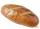# Sumo competition

In the competition for the heaviest man was 5 competitors. The first three together weighed 553 kg. What is the minimal weigt of winner, if a contestant weighed with an accuracy of one kilogram.

Correct result:

m1min =  185 kg

#### Solution:Our examples were largely sent or created by pupils and students themselves. Therefore, we would be pleased if you could send us any errors you found, spelling mistakes, or rephasing the example. Thank you!

Leave us a comment of this math problem and its solution (i.e. if it is still somewhat unclear...):Be the first to comment!Tips to related online calculators
Do you have a linear equation or system of equations and looking for its solution? Or do you have quadratic equation?

## Next similar math problems:

• NormThree workers planted 3555 seedlings of tomatoes in one dey. First worked at the standard norm, the second planted 120 seedlings more and the third 135 seedlings more than the first worker. How many seedlings were standard norm?5 of the same bread has the same weight as three bread and 4 kg of fruit. What weight has one bread?
• Unknown number 11That number increased by three equals three times itself?
• Dropped sheetsThree consecutive sheets dropped from the book. The sum of the numbers on the pages of the dropped sheets is 273. What number has the last page of the dropped sheets?
• Simple equationSolve the following simple equation: 2. (4x + 3) = 2-5. (1-x)
• Simple equationSolve for x: 3(x + 2) = x - 18
• Simple equation 1035= 7*3*x what is x?
• Equation 29Solve next equation: 2 ( 2x + 3 ) = 8 ( 1 - x) -5 ( x -2 )
• Tickets 3A total of 645 tickets were sold for the school play. They were either adult tickets or student tickets. There were 55 fewer student tickets sold than adult tickets. How many adult tickets were sold?
• Negative in equation2x + 3 + 7x = – 24, what is the value of x?
• Forest nurseryIn the forest nursery after winter, they found that 1/10 stems died out of them. For them, they land 193 new spruces. How many spruces are in the forest nursery?
• Find xSolve: if 2(x-1)=14, then x= (solve an equation with one unknown)
• Simple equation 8Solve the following equation: 36=-(1+7x)-6(-7-x)
• InfirmaryTwo thirds of children from the infirmary went on a trip seventh went to bathe and 40 children remained in the gym. How many children were treated in the infirmary?
• The math testThe math test contains 20 problems. For each correctly solved problem, the solver gets 3 points, for each incorrectly solved or unsolved problem, 2 points are deducted. Ondrej got 25 points. How many problems did he solve correctly?
• Simple equation 9Solve the following equation: -8y+5=-9y+9
• SuzanSusan's age will be after 12 years four times as much as twelve years ago. How old is Susan now?